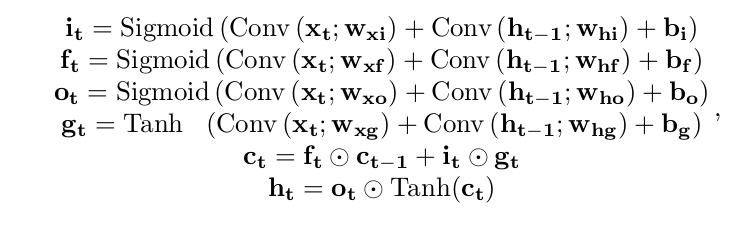Need help with ConvLSTM_pytorch?
Click the “chat” button below for chat support from the developer who created it, or find similar developers for support.ndrplz944 Stars303 ForksMIT License11 Commits15 Opened issues#### Description

Implementation of Convolutional LSTM in PyTorch.!
?

#### Need anything else?# ConvLSTM_pytorch

This file contains the implementation of Convolutional LSTM in PyTorch made by me and DavideA.

We started from this implementation and heavily refactored it add added features to match our needs.

Please note that in this repository we implement the following dynamics:which is a bit different from the one in the original paper.

### How to Use

The

`ConvLSTM`
module derives from
`nn.Module`
so it can be used as any other PyTorch module.

The ConvLSTM class supports an arbitrary number of layers. In this case, it can be specified the hidden dimension (that is, the number of channels) and the kernel size of each layer. In the case more layers are present but a single value is provided, this is replicated for all the layers. For example, in the following snippet each of the three layers has a different hidden dimension but the same kernel size.

Example usage:

```model = ConvLSTM(input_dim=channels,
hidden_dim=[64, 64, 128],
kernel_size=(3, 3),
num_layers=3,
batch_first=True
bias=True,
return_all_layers=False)
```

• Comment code